International
Tables for
Crystallography
Volume H
Powder diffraction
Edited by C. J. Gilmore, J. A. Kaduk and H. Schenk

International Tables for Crystallography (2018). Vol. H, ch. 3.8, pp. 337-339

## Section 3.8.7. Quantitative analysis with high-throughput PXRD data without Rietveld refinement

C. J. Gilmore,a G. Barra and W. Donga*

aDepartment of Chemistry, University of Glasgow, University Avenue, Glasgow, G12 8QQ, UK
Correspondence e-mail:  chris@chem.gla.ac.uk

### 3.8.7. Quantitative analysis with high-throughput PXRD data without Rietveld refinement

| top | pdf |

Since mixtures are so common in high-throughput experiments, and indeed in many situations with multiple data sets, it is useful to have a method of automatic quantitative analysis. The quality of data that results from high-throughput crystallography makes it unlikely that an accuracy better than 5–10% can be achieved but, nonetheless, the identification of mixtures can be carried out by whole-profile matching. First a database of N pure phases is created, or, if that is not possible, then the most representative patterns with appropriate safeguards can be used. Assume that there is a sample pattern, S, which is considered to be a mixture of up to N components. S comprises m data points, S1, S2, …, Sm. The N patterns can be considered to make up fractions p1, p2, p3, …, pN of the sample pattern. The best possible combination of the database patterns to fit the sample pattern is required. A system of linear equations can be constructed in which x11 is measurement point 1 of pattern 1 etc.: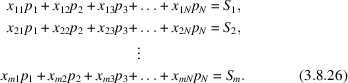Writing these in matrix form, we get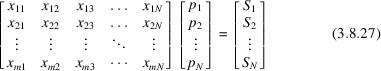or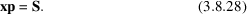A solution for S that minimizes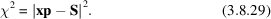is required. Since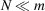, the system is heavily overdetermined, and least-squares or singular value decomposition can be used to solve (3.8.29)for the fractional percentages arising from the scattering power of the component mixtures,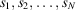. The values of s can be used to calculate a weight fraction for that particular phase provided that the atomic absorption coefficients are known, and this in turn requires the unit-cell dimensions and cell contents, but not the atomic coordinates (Smith et al., 1988; Cressey & Schofield, 1996). The general formula for the weight fraction of component n in a mixture comprising N components is (Leroux et al., 1953)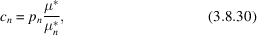where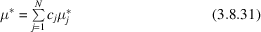and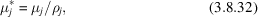where μj is the atomic X-ray absorption coefficient and ρj is the density of component j. For polymorphs, the absorption coefficients are sufficiently close and the method sufficiently approximate that the effects of absorption can be ignored.

#### 3.8.7.1. Example: inorganic mixtures

| top | pdf |

As an example, a set of 19 patterns from set 78 of the ICDD database for inorganic compounds (ICDD, 2018) was imported into DIFFRAC.EVA. To this was added some simulated mixture data generated by adding the patterns for lanthanum strontium copper oxide and caesium thiocyanate in the proportions 80/20, 60/40, 50/50, 40/60 and 20/80. Two calculations were performed: an analysis without the pure-phase database and a second where the pure phases of lanthanum strontium copper oxide and caesium thiocyanate were present.

The results are shown in Fig. 3.8.12. In the MMDS plot the green spheres represent pure lanthanum strontium copper oxide while the yellow are pure caesium thiocyanate. The red spheres represent mixtures of the two. The latter form an arc between the green and yellow clusters. The distance of the spheres representing mixtures from the lanthanum strontium copper oxide and caesium thiocyanate spheres gives a semi-quantitative representation of the mixture contents. Running the analysis in quantitative mode gives the pie charts also shown in Fig. 3.8.12; they reproduce exactly the relative proportions of the three components.Figure 3.8.12 | top | pdf |Identifying mixtures using lanthanum strontium copper oxide and caesium thiocyanate diffraction data taken from the ICDD Clay Minerals database. The green spheres represent pure phases of lanthanum strontium copper oxide and the yellow pure caesium thiocyanate. The red spheres represent mixtures of the two in the relative proportions of lanthanum strontium copper oxide/caesium thiocyanate 80/20, 60/40, 50/50, 40/60 and 20/80 in an arc commencing on the left-hand side of the diagram. The pie charts give the results of an independent quantitative calculation in which lanthanum strontium copper oxide and caesium thiocyanate have been included as pure phases in a reference database.

For further details of this method with organic samples, see Dong et al. (2008).

### References

Cressey, G. & Schofield, P. F. (1996). Rapid whole-pattern profile-stripping method for the quantification of multiphase samples. Powder Diffr. 11, 35–39.Google Scholar
Dong, W., Gilmore, C., Barr, G., Dallman, C., Feeder, N. & Terry, S. (2008). A quick method for the quantitative analysis of mixtures. 1. Powder X-ray diffraction. J. Pharm. Sci. 97, 2260–2276.Google Scholar
ICDD (2018). The Powder Diffraction File. International Centre for Diffraction Data, 12 Campus Boulevard, Newton Square, Pennsylvania 19073-3273, USA.Google Scholar
Leroux, J., Lennox, D. H. & Kay, K. (1953). Direct quantitative X-ray analysis by diffraction absorption technique. Anal. Chem. 25, 740–743.Google Scholar
Smith, D. K., Johnson, G. G. & Wims, A. M. (1988). Use of full diffraction spectra, both experimental and calculated, in quantitative powder diffraction analysis. Aust. J. Phys. 41, 311–321.Google Scholar